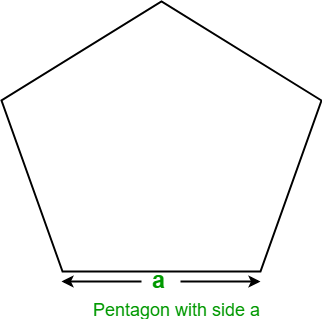# Program to find the Area of Pentagon

• Last Updated : 27 Aug, 2022

Given the side of a Pentagon, the task is to find the area of the Pentagon.
Examples:

Input : a = 5
Output: Area of Pentagon: 43.0119

Input : a = 10
Output: Area of Pentagon: 172.047745

A regular pentagon is a five sided geometric shape whose all sides and angles are equal. Its interior angles are of 108 degrees each and its exterior angles are 72 degrees each. The sum of interior angles of a pentagon is 540 degrees.Let a be the side of Pentagon, then the formula to find the area of Pentagon given by
Area =## C++

 // C++ program to find the area of Pentagon #include using namespace std;   // Function to find area of pentagon float findArea(float a) {     float area;         // Formula to find area     area = (sqrt(5 * (5 + 2 * (sqrt(5)))) * a * a) / 4;         return area; }   // Driver code int main() {     float a = 5;           // function calling     cout << "Area of Pentagon: " << findArea(a);           return 0; }

## Java

 // Java program to find the area of Pentagon import java.io.*;   class GFG {           // Function to find area of pentagon     static float findArea(float a)     {         float area;                 // Formula to find area         area = (float)(Math.sqrt(5 * (5 + 2                 * (Math.sqrt(5)))) * a * a) / 4;                 return area;     }           // Driver code     public static void main (String[] args)      {         float a = 5;                   System.out.println("Area of Pentagon: "                                + findArea(a));     } }

## Python3

 # Python3 program to find  # the area of Pentagon   # Import Math module # to use sqrt function from math import sqrt   # Function to find  # area of pentagon   def findArea(a):           # Formula to find area     area = (sqrt(5 * (5 + 2 *            (sqrt(5)))) * a * a) / 4     return area         # Driver code a = 5   # call function findArea() # to calculate area of pentagon # and print the calculated area print("Area of Pentagon: ",                 findArea(a))    # This code is contributed  # by ihritik

## C#

 // C# program to find  // the area of Pentagon using System;   class GFG  {           // Function to find      // area of pentagon     static float findArea(float a)     {         float area;               // Formula to find area         area = (float)(Math.Sqrt(5 * (5 + 2 *                        (Math.Sqrt(5)))) *                               a * a) / 4;               return area;     }           // Driver code     public static void Main ()      {         float a = 5;                   Console.WriteLine("Area of Pentagon: "+                                    findArea(a));     } }   // This code is contributed  // by anuj_67.

## PHP

 

## Javascript

 

Output:

Area of Pentagon: 43.0119

Time complexity: O(1)

Auxiliary Space: O(1), since no extra space has been taken.

My Personal Notes arrow_drop_up
Recommended Articles
Page :